# How to shuffle a range in Google Sheets

Tuesday, February 23, 2021

Spreadsheets don't have to be organized all of the time. Sometimes, you need a little chaos.

Google Sheets provides a few ways to introduce randomness into your rows, like the `RAND()` formula, or Randomize Range in the Data menu, but these are limited. For example, `RAND()` can't guarantee that there won't be duplicates across multiple cells, and Randomize Range only works for columns (and you have to activate it manually, at that).

What if I have a row of values already set, and just want to shuffle the cells around? For example, a list of chores to be assigned randomly, or an event that needs to happen on a different day each week? What if you just want to shuffle a virtual deck of cards?

This feels like something that should be easy, but it can prove complicated if you don't have your data set up in the way Google expects. Luckily, we can shuffle a range with a single formula. It just takes a little trickery with the way Google Sheets thinks about rows, columns, and arrays.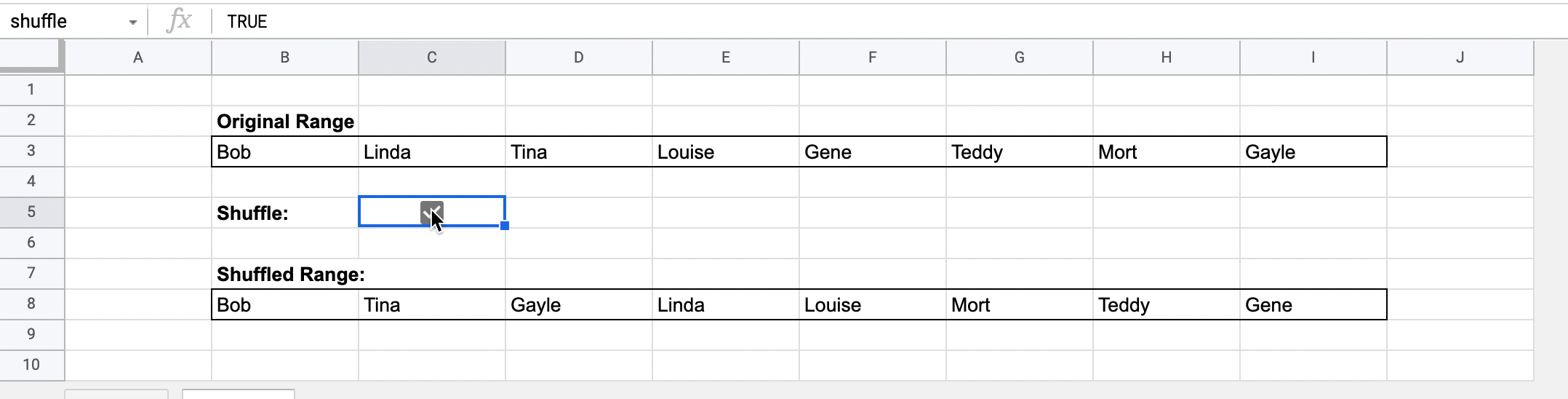## Setting up the pieces

To shuffle our range of cells, we'll start with the static list of values. In the example above, these are a list of names, kept in the `B3:I3` range.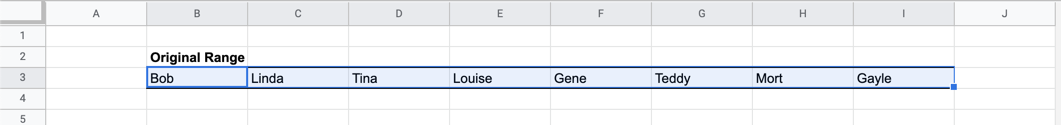These cells will stay static, though you can update their values at any time.

To shuffle them, we'll want to temporarily turn the row into a column, with the `TRANSPOSE()` formula. That turns rows into columns, and vice-versa. We're actually going to use it twice in our formula, to turn the values back into a row when we're done. For now, we want a column.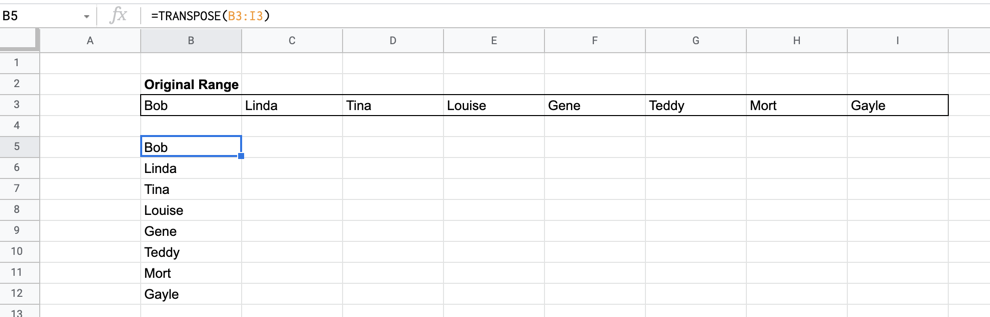Then, we want to match each of those values up with a random number. We can do that by putting a `RANDARRAY()` formula into the next column over. `RANDARRAY()` is similar to `RAND()` in that it gives us a random number between 0 and 1, but it can repeat that process across a number of rows and columns. Because we only have one column of names, we know we want one of those, and we can use a `COUNTA()` formula to get the number of rows. That ends up looking like this:

`=RANDARRAY(COUNTA(B3:I3),1)`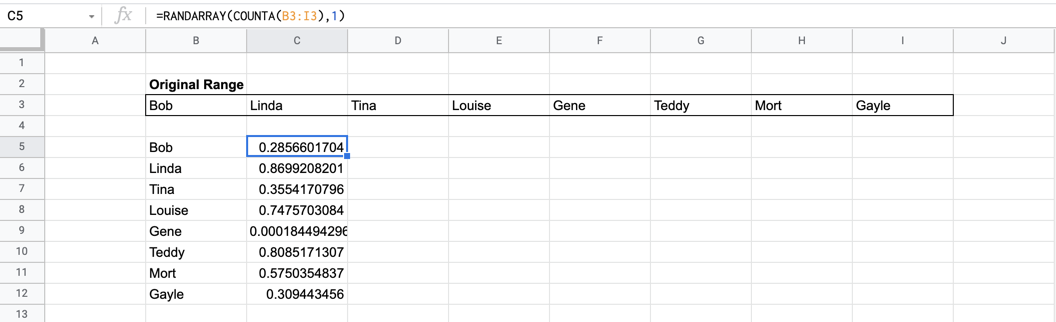And here's where the shuffling comes in: if we sort the column of names based on the column of numbers, we can return the names in a random order! The simplest version of this is the `SORT()` formula: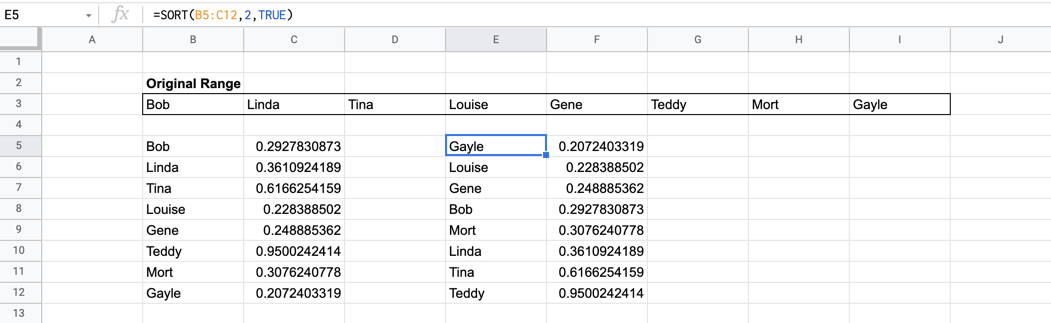But I only want the names, so I'll turn this into a `QUERY()` instead.

`=QUERY(B5:C12,"SELECT B ORDER BY C")`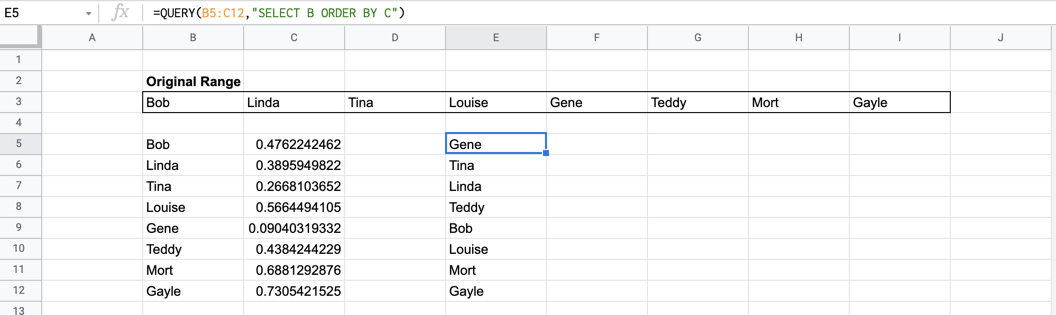Using the `QUERY()` formula's ORDER BY rule, we can sort the data using the second column, without a `SORT()` formula. And using SELECT lets us grab just the first column from the result. (You can read more about QUERY() here, it's great.)

We can bring back another `TRANSPOSE()` to turn the values back into a row.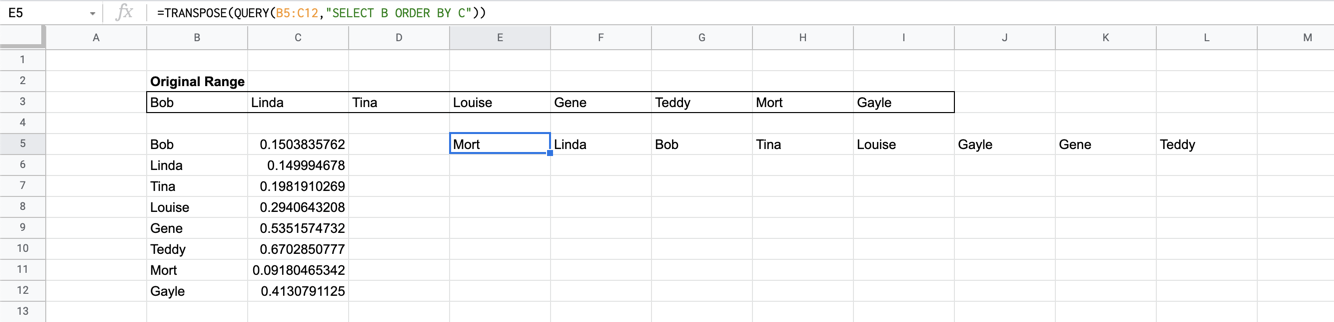## Tidying up

While it works, that setup looks a little messy—I don't want to have a big chunk with random numbers in my spreadsheet! To clean things up a bit, we're going to abstract out those first few steps, by putting them into an array.

Instead of putting our `TRANSPOSE()` and `RANDARRAY()` formulas into two separate cells, we can combine them into a single formula by wrapping them in { brackets }, like this:

`={TRANSPOSE(B3:I3),RANDARRAY(COUNTA(B3:I3),1)}`

When Google Sheets receives a bracketed value like that, it treats each argument (separated by commas) as a separate column, and displays them accordingly. Replacing those formulas lets our example look pretty much the same, but with one fewer calculation in the mix.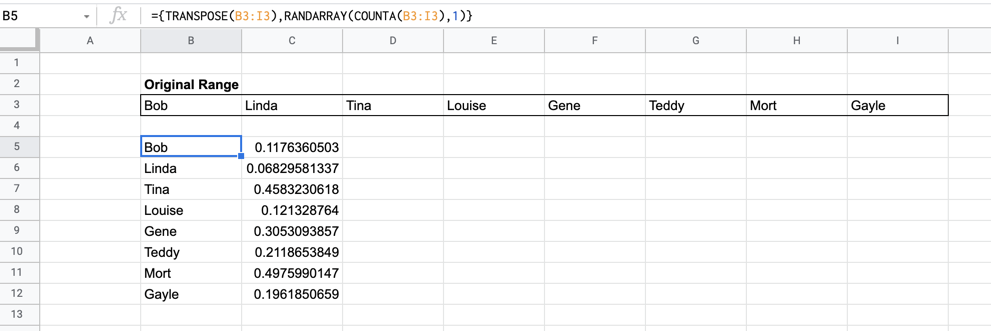And for bonus points, we don't even need to put that in a separate cell! We can pop it straight into the `QUERY()` as its data, and wrap the whole thing in a `TRANSPOSE()` so that the result of the query is displayed in a row.

`=TRANSPOSE(QUERY({TRANSPOSE(B3:I3),RANDARRAY(COUNTA(B3:I3),1)}, "SELECT Col1 ORDER BY Col2"))`

When we abstract arrays in this way, all of our formulas work the same, but their results don't actually exist on the spreadsheet anymore. They only exist in the middle of the formula, if you will, so we can't use column names like A, B, or C in our query.

Instead of `SELECT B ORDER BY C`, we use `Col1` and `Col2` to identify which column we want to display, and which column to use for ordering. Since the second argument in our bracketed array is a list of random numbers, we use that to sort by, and return the first column (our original set of names) in that order.

Now you have a shuffled range of values, neatly tucked away in a single formula!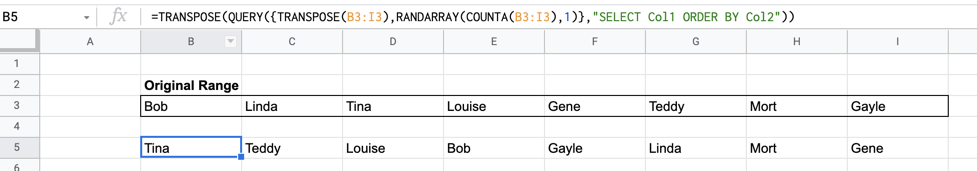## Shuffling on command

Y'all know me, I can't make a spreadsheet without turning on iterative calculation. In this case, it lets us turn the shuffling off and on, too! To set it up, head to File > Spreadsheet Settings > Calculation and turn on Iterative calculation. This lets cells reference themselves in formulas, and I tend to set Max. number of iterations to 1.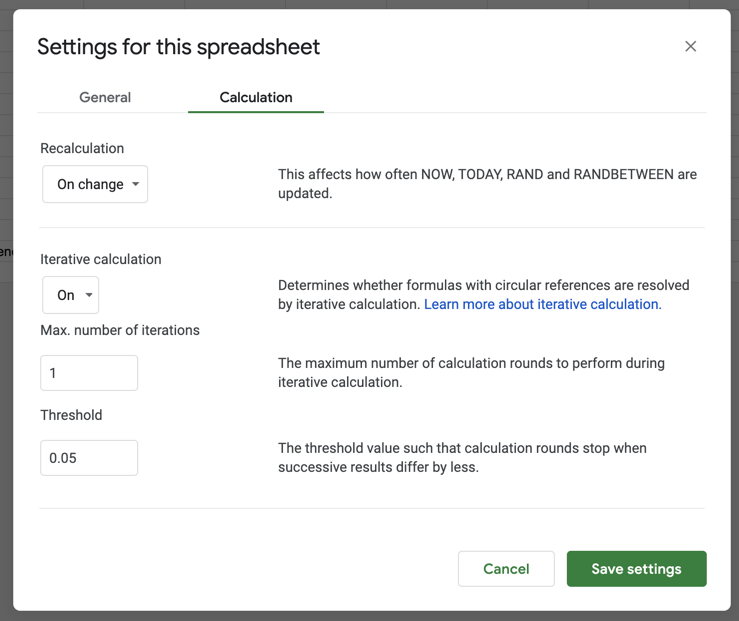Then, let's pop a checkbox into a cell, and use Named Ranges to call it `shuffle`. We can add an `IF()` to our formula from before, so that it only shuffles when that checkbox is checked, and stays as-is otherwise.

`=IF(shuffle,TRANSPOSE(QUERY({TRANSPOSE(B3:I3),RANDARRAY(COUNTA(B3:I3),1)},"SELECT Col1 ORDER BY Col2")),B8:I8)`## What do you think?

Do you have a use for this? Is there a simpler method that I've overlooked? What other ways can we use `QUERY()` to make this process more useful?